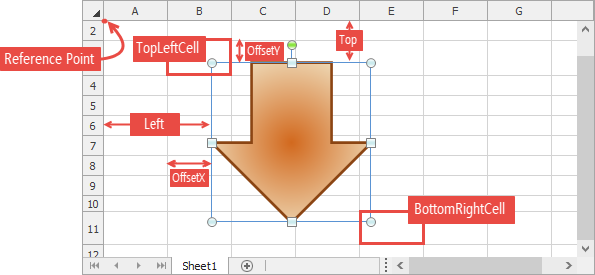## End-User Documentation

19.2
19.1
18.2
18.1
17.2
.NET Framework 4.5.2+
.NET Framework 4.5.2+
.NET Standard 2.0+
.NET Core 3.0+

# FloatingObject.Move(Single, Single) Method

Moves a floating object by a specified offset.

## Declaration

``````void Move(
float rowOffset,
float columnOffset
)``````
``````Sub Move(
rowOffset As Single,
columnOffset As Single
)``````
``````void Move(
float rowOffset,
float columnOffset
)``````
``````Sub Move(
rowOffset As Single,
columnOffset As Single
)``````
``````void Move(
float rowOffset,
float columnOffset
)``````
``````Sub Move(
rowOffset As Single,
columnOffset As Single
)``````

## Parameters

Name Type Description
rowOffset Single

A Single that is the horizontal (column) offset measured in units specified by the ISpreadsheetComponent.Unit property.

columnOffset Single

A Single that is the vertical (row) offset measured in units specified by the ISpreadsheetComponent.Unit property.

## Parameters

Name Type Description
rowOffset Single

A Single that is the horizontal (column) offset measured in units specified by the ISpreadsheetComponent.Unit property.

columnOffset Single

A Single that is the vertical (row) offset measured in units specified by the ISpreadsheetComponent.Unit property.

## Parameters

Name Type Description
rowOffset Single

A Single that is the horizontal (column) offset measured in units specified by the ISpreadsheetComponent.Unit property.

columnOffset Single

A Single that is the vertical (row) offset measured in units specified by the ISpreadsheetComponent.Unit property.

## Remarks

The image below illustrates the distances used to specify a picture's placement on a worksheet.## Examples

##### Note

A complete sample project is available at https://github.com/DevExpress-Examples/winforms-spreadsheetcontrol-api-part-2-e4832

``````' Set the measurement unit to Millimeter.
workbook.Unit = DevExpress.Office.DocumentUnit.Millimeter
workbook.Worksheets.ActiveWorksheet.DefaultRowHeight = 20
workbook.Worksheets.ActiveWorksheet.DefaultColumnWidth = 20

workbook.BeginUpdate()
Try
Dim worksheet As Worksheet = workbook.Worksheets(0)
' Insert pictures.
' Specify picture name.
pic.Name = "Logo"